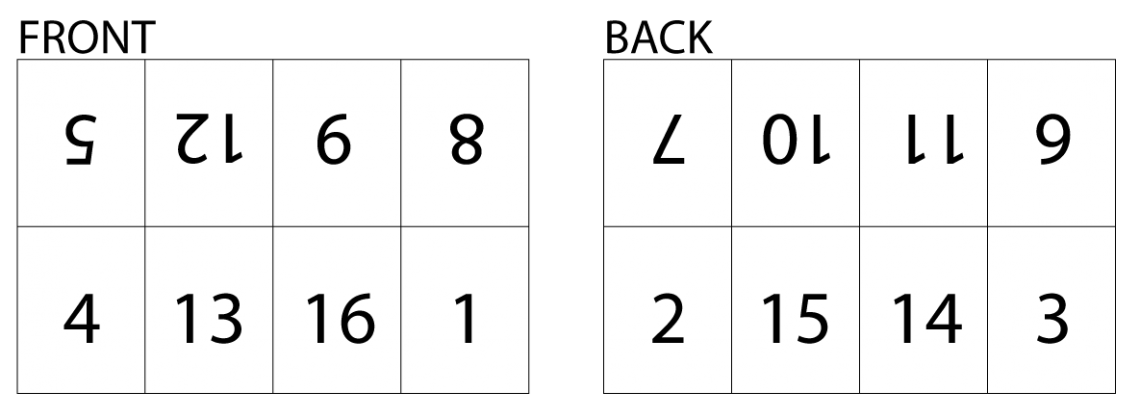How to convert A3 PDFs into a booklet that will fold when printed.

I need a variation on the example given in answer to the following question: How to convert an existing A4 PDF document to an A3 booklet? I have more or less the same requirement, but I don't want the consecutive pages to appear side by side on the same page. I want page 4 and 1 on one page and page 2 and 3 on another page. So, when I print these pages on single sheet of paper, I can fold that paper into a booklet with page 2 on the back of page 1 and page 4 on the back of page 3.

Posted on StackOverflow on Dec 23, 2015 by Anil Kumar

When a book is printed, the machines that print the pages actually print on much larger pages, and that larger page is then usually cut and folded. You'll see that most books have a total number of pages that is dividable by 16. For instance, when I wrote my "iText in Action" books, extra pages with advertisements for other books were added so that the total number of pages would be a multiple of 16.

This shows how pages are typically printed:How book pages are printed

(The image was taken from a blog entitled "Page counts explained")

We can easily convert a document with A4 pages to a document with pages 8 times the size of A4, where we add 8 pages of the original document to a single page in the new document, organized the way is shown in the above image. This is done in the MakeBooklet example:

``````public void manipulatePdf(String src, String dest) throws IOException {
PdfDocument srcDoc = new PdfDocument(new PdfReader(src));
PdfDocument pdfDoc = new PdfDocument(new PdfWriter(dest));

float a4_width = PageSize.A4.getWidth();
float a4_height = PageSize.A4.getHeight();
PageSize pagesize = new PageSize(a4_width * 4, a4_height * 2);
pdfDoc.setDefaultPageSize(pagesize);

int n = srcDoc.getNumberOfPages();
int p = 1;
while ((p - 1) / 16  srcDoc.getNumberOfPages()) {
return;
}
PdfFormXObject page = srcDoc.getPage(p).copyAsFormXObject(pdfDoc);
}
``````

In the `manipulatePdf()` method, we create a loop in which we add 2 pages. On each page, we add 8 pages of the original PDF, but we add these on very specific places. For the bottom row of 4 pages, we introduce a translation (we move `a4_width` to the right for every new page). Then we introduce a rotation to add the top row of pages (they need to be upside-down).

That's fun isn't it? Actually, it's just a matter of Math (and Math is always fun). You can find the result here: book16.pdf

Your question is less fun because it's so simple. You don't even need to rotate your pages! Take a look at the MakeBookletA3 example:

``````public void manipulatePdf(String src, String dest) throws IOException {
PdfDocument srcDoc = new PdfDocument(new PdfReader(src));
PdfDocument pdfDoc = new PdfDocument(new PdfWriter(dest));
PageSize pageSize = new PageSize(
PageSize.A4.getWidth() * 2,
PageSize.A4.getHeight());
pdfDoc.setDefaultPageSize(pageSize);
float a4_width = PageSize.A4.getWidth();
int n = srcDoc.getNumberOfPages();
int p = 1;
while ((p - 1) / 4  srcDoc.getNumberOfPages()) {
return;
}
PdfFormXObject page = srcDoc.getPage(p).copyAsFormXObject(pdfDoc);